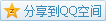# MIT OCW

## 18.02 Multivariable Calculus

### Substitution of Variables

#### Introduction

e.g. Calculate the area of a ellipse $\left(\frac{x}{a}\right)^2+\left(\frac{y}{b}\right)^2=1$ with substitution $u=\frac{x}{a},v=\frac{y}{b}$.
Solution From the substitution, we can find that $$\mathrm{d}x=a\mathrm{d}u,\mathrm{d}y=b\mathrm{d}v,$$
so we can convert it
\begin{aligned}\iint_R\mathrm{d}x\mathrm{dy}&=ab\iint_{R'}\mathrm{d}u\mathrm{d}v\\&=ab\iint_{u^2+v^2\leq 1}\mathrm{d}u\mathrm{d}v\\&=\pi ab.\end{aligned}
e.g. Find the scaling factor between $\mathrm{d}x\mathrm{d}y$ and $\mathrm{d}u\mathrm{d}v$ with $u=3x-2y,v=x+y$.
Solution We can write such transformation in matrix form $$\begin{bmatrix}u\\v\end{bmatrix}=\begin{bmatrix}3&-2\\1&1\end{bmatrix}\begin{bmatrix}x\\y\end{bmatrix}.$$
And note the core here is the matrix $\begin{bmatrix}3&-2\\1&1\end{bmatrix}$, it represents the transformation and its determinant is just the scaling factor but with a sign. So the scaling factor here is $\begin{vmatrix}3&-2\\1&1\end{vmatrix}=5$.

#### Jacobian Matrix

The total differential of a transform $u=u(x,y),v=v(x,y)$ is \begin{aligned}\mathrm{d}u&=u_{x}\mathrm{d}x+u_{y}\mathrm{d}y\\\mathrm{d}v&=v_{x}\mathrm{d}x+u_{v}\mathrm{d}y,\end{aligned}
and write it in matrix form $$\begin{bmatrix}\mathrm{d}u\\\mathrm{d}v\end{bmatrix}=\begin{bmatrix}u_x&u_y\\v_x&v_y\end{bmatrix}\begin{bmatrix}\mathrm{d}x\\\mathrm{d}y\end{bmatrix}.$$
Here, the matrix containing several partial derivatives are called Jacobian matrix, and usually write it as $$\mathbf{J}=\frac{\partial(u,v)}{\partial(x,y)}=\begin{bmatrix}u_x&u_y\\v_x&v_y\end{bmatrix}.$$
And its determinant $|\mathbf{J}|$ is the scaling factor.

#### Validation for Polar-Cartesian Conversion

The transformation here is $x=r\cos(\theta),y=r\sin(\theta)$, the Jacobian determinant is $$\left|\frac{\partial(x,y)}{\partial(r,\theta)}\right|=\begin{vmatrix}x_r&x_\theta\\y_r&y_\theta\end{vmatrix}=\begin{vmatrix}\cos(\theta)&-r\sin(\theta)\\\sin(\theta)&r\cos(\theta)\end{vmatrix}=r,$$
so we have $$\mathrm{d}x\mathrm{d}y=r\mathrm{d}r\mathrm{d}\theta.$$

#### Examples

e.g. Compute $\int_0^1\int_0^1x^2y\mathrm{d}x\mathrm{d}y$ with substitution $u=x,v=xy$.
Solution The Jacobian determinant is $$\left|\frac{\partial(u,v)}{\partial(x,y)}\right|=\begin{vmatrix}u_x&u_y\\v_x&v_y\end{vmatrix}=\begin{vmatrix}1&0\\y&x\end{vmatrix}=x,$$
therefore we have $$\mathrm{d}x\mathrm{d}y=\frac1x\mathrm{d}u\mathrm{d}v.$$
Another thing to consider is the new region, according to the substitution we have $v=y\cdot u$.
In an $uv$ plane, $v=y\cdot u, u\in[0,1]$ is a segment through the origin, and $y$ becomes its slope, and it varies since $y\in[0,1]$, so it becomes a triangular region.
Here's the comparison of two regions.So the integral becomes \begin{aligned}\int_0^1\int_0^1x^2y\mathrm{d}x\mathrm{d}y&=\iint_{R'}x^2y\frac1x\mathrm{d}u\mathrm{d}v\\&=\iint_{R'}v\mathrm{d}v\mathrm{d}u\\&=\int_0^1\int_0^{u}v\mathrm{d}v\mathrm{d}u\\&=\int_0^1\left[\frac12v^2\right]^u_0\mathrm{d}u\\&=\int_0^1\frac12u^2\mathrm{d}u\\&=\left[\frac16u^3\right]^1_0\\&=\frac16.\end{aligned}
Since the original integral is not difficult to compute, so the answer can be checked easily.